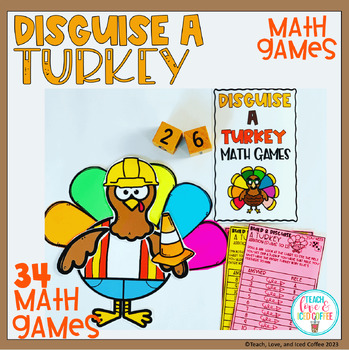# Disguise A Turkey Math GamesSubject
Resource Type
Format
Zip (127 MB|90+)
Standards
\$3.60
List Price:
\$4.50
You Save:
\$0.90
\$3.60
List Price:
\$4.50
You Save:
\$0.90

### Description

These Turkeys Are Too Cute To Eat!

There Are 32 Math Games To Play In This Set. There's Addition, Subtraction, And Multiplication. Games Are Listed Below.

There Are 28 ADORABLE Turkeys For Your Kids To Build And Disguise While They Are Practicing Math Skills.

This Resource Is Fun And Engaging.

Use For Early Finishers Or For Differentiation.

Warning: This Takes Some Time To Prep. So Keep That In Mind Before You Purchase. Also, The Turkey Body Parts Are In Color Only. The Feathers Are In Both Color And Black And White. My Other Math Building Games Have Lasted Me for Years.

Games Included:

• Sums to 12
• Sums to 20
• Doubles 1- 6
• Doubles 7-12
• Doubles 0-10
• Make 10
• Plus 10
• Odd/even 1-6
• Odd/even 1-10
• Add 2 numbers & odd/even
• Add 3 numbers & odd/even

SUBTRACTION

• Subtract from 6
• Subtract 1-6 from 10
• Subtract from 10
• Subtract 1-10 from 20

MULTIPLICATION

• Multiply 1-10 by 1
• Multiply 1-10 by 2
• Multiply 1-10 by 3
• Multiply 1-10 by 4
• Multiply 1-10 by 5
• Multiply 1-10 by 6
• Multiply 1-10 by 7
• Multiply 1-10 by 8
• Multiply 1-10 by 9
• Multiply 1-10 by 10
• Multiply 1, 2, 3 to 10
• Multiply 4, 5, 6 to 10
• Multiply 7, 8, 9 to 10
Total Pages
90+
N/A
Teaching Duration
N/A
Report this Resource to TpT
Reported resources will be reviewed by our team. Report this resource to let us know if this resource violates TpT’s content guidelines.

### Standards

to see state-specific standards (only available in the US).
Interpret products of whole numbers, e.g., interpret 5 × 7 as the total number of objects in 5 groups of 7 objects each. For example, describe a context in which a total number of objects can be expressed as 5 × 7.
Fluently add and subtract within 20 using mental strategies. By end of Grade 2, know from memory all sums of two one-digit numbers.
Add and subtract within 20, demonstrating fluency for addition and subtraction within 10. Use strategies such as counting on; making ten (e.g., 8 + 6 = 8 + 2 + 4 = 10 + 4 = 14); decomposing a number leading to a ten (e.g., 13 - 4 = 13 - 3 - 1 = 10 - 1 = 9); using the relationship between addition and subtraction (e.g., knowing that 8 + 4 = 12, one knows 12 - 8 = 4); and creating equivalent but easier or known sums (e.g., adding 6 + 7 by creating the known equivalent 6 + 6 + 1 = 12 + 1 = 13).